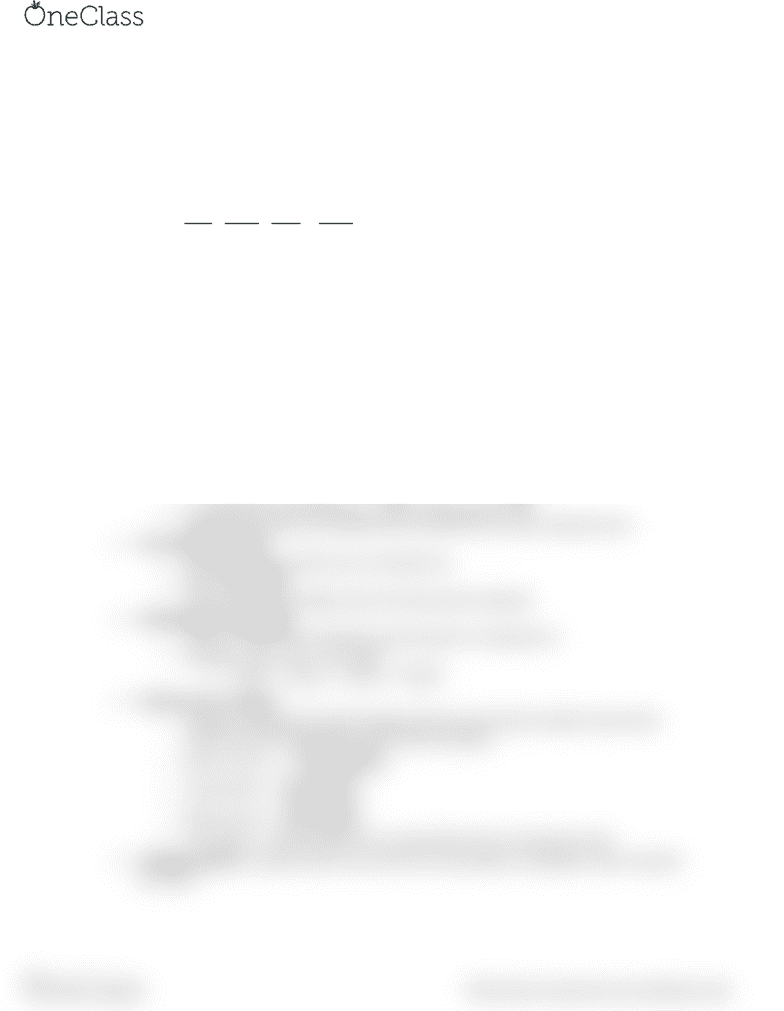Study Guides (380,000)
CA (150,000)
UW (6,000)
CHE (30)
CHE102 (8)
Final

CHE102 Study Guide - Final Guide: Partial Pressure, Gas Constant, Ideal Gas Law

Department
Chemical Engineering
Course Code
CHE102
Professor
Perry Chou
Study Guide
Final

This preview shows half of the first page. to view the full 2 pages of the document.CHE 102 Final Notes
One mol of a compound means that there is 6.022*10^23 atoms of that compound
o Periodic table shows us the grams/mol of each element (very useful for solving
questions)
For unit conversions a method is to list it in the following manner (example converting 100m/s
to km/h):
o 
 

 

o The unit meters and seconds would cancel out and we are left with hours and
kilometers
Classification of matter
o Pure Substance (can’t e separated y physial proess)
Compound (2 or more elements)
Elements (on periodic table)
o Mixture (can be separated by physical process
Homogeneous (one phase)
Heterogeneous (multiple phases)
1amu = 1g/NA = 1/6.022*10^23 g (NA=avogadro’s uer)
Empirical + Molecular formulas
o Empirical formula shows us partial information, telling us relative number of atoms in a
molecule and many diff substances may have same empirical formula
o Molecular formula shows us actual # of atoms in a molecule
o i.e molecular formula of hexane -> C6H12 and empirical -> CH2
o Molecular formula can be obtained from empirical if we know molecular mass
The chemical equation
o Describes changes which occur in chemical rxn
o Must be balanced
o Only shows overall changes, does not show steps in between
Stoichiometric calculations
o Shows us proportions of products and reactants in a chemical rxn
o Example -> CH4 + 2O2 -> CO2 +2H2O




Chemical rxn in solution
o Solutions composed of solvents (largest amount) and solutes (smaller amount than
solvent) and are homogeneous with uniform property
o   

o 

o 

o Percentage -> volume/volume (v/v), mass/volume (w/v), mass/mass (w/w)
Limiting reactant is reactant which runs out first if rxn proceeds to completion, other reactants
are excess
find more resources at oneclass.com
find more resources at oneclass.com